Question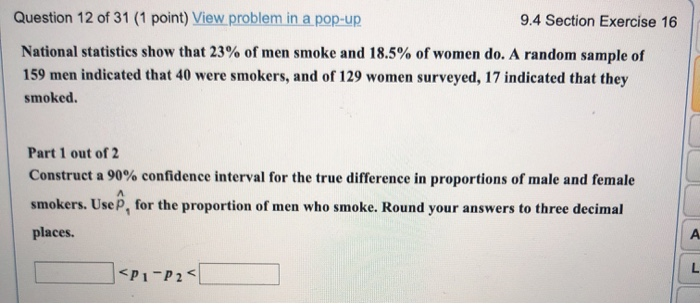Question 12) Given that,

For men : n1 = 159 and x1 = 40

For women : n2 = 129 and x2 = 17

Using TI-83 plus calculator we get, the 90% confidence interval for the true difference in proportions of male and female smokers is,

0.045 < p1 - p2 < 0.195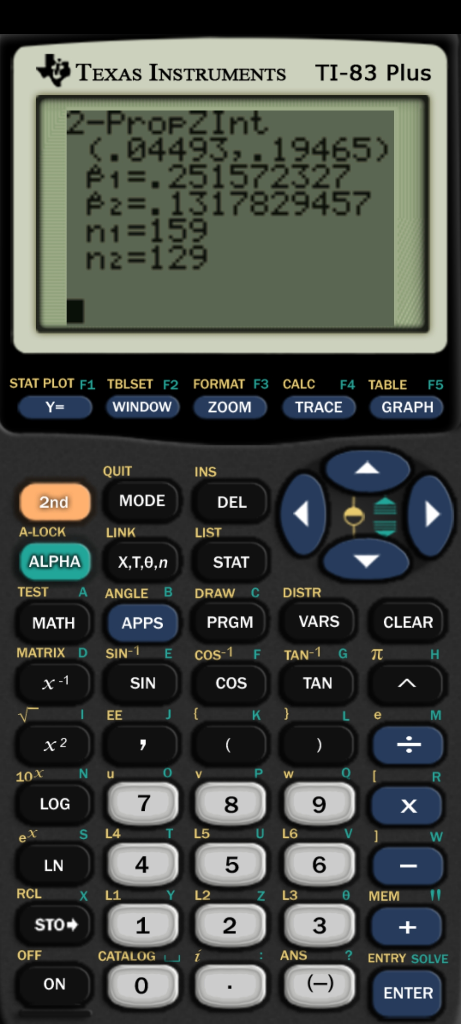#### Earn Coins

Coins can be redeemed for fabulous gifts.

Similar Homework Help Questions
• ### Question 11 of 31 (1 point) 9.4 Section Exercise 15 (table) A recent random survey of...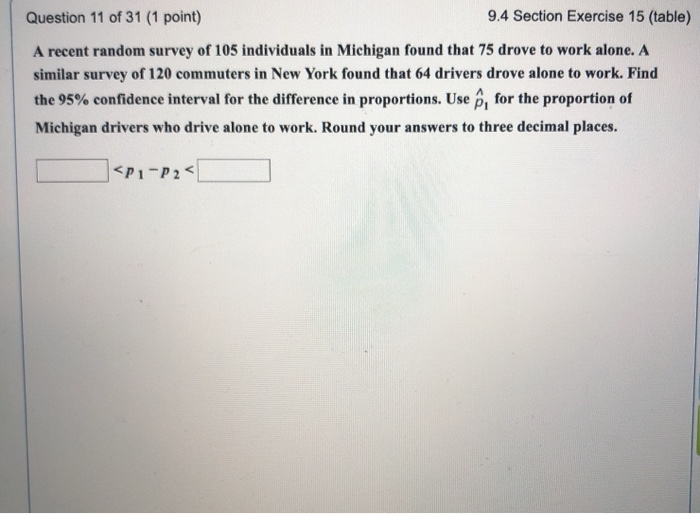Question 11 of 31 (1 point) 9.4 Section Exercise 15 (table) A recent random survey of 105 individuals in Michigan found that 75 drove to work alone. A similar survey of 120 commuters in New York found that 64 drivers drove alone to work. Find the 95% confidence interval for the difference in proportions. Use ê, for the proportion of Michigan drivers who drive alone to work. Round your answers to three decimal places. <P1-P2 Question 12 of 31 (1...

• ### Question 12 of 19 (1 point) View problem in a pop-up 10.2 Section Exercise 13 Question...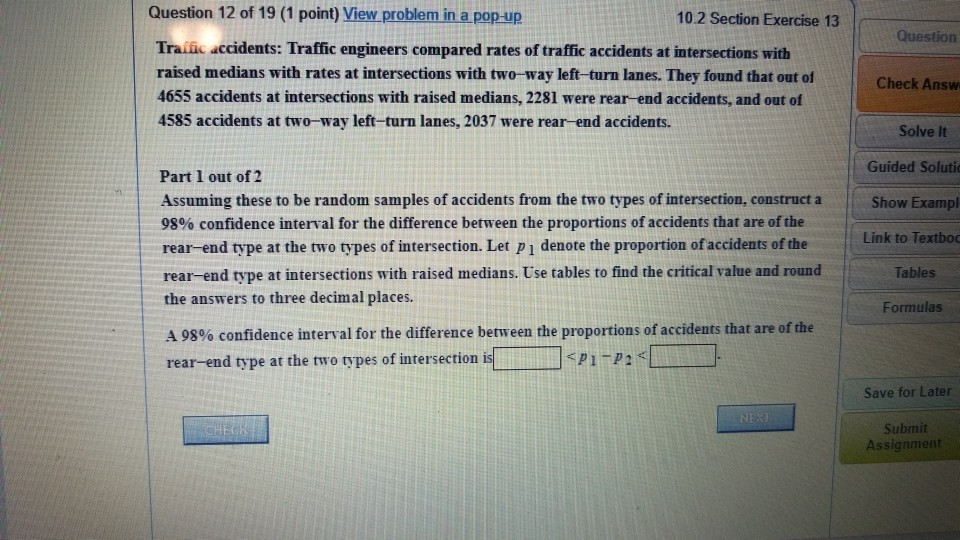Question 12 of 19 (1 point) View problem in a pop-up 10.2 Section Exercise 13 Question Traiic accidents: Traffic engineers compared rates of traffic accidents at intersections with raised medians with rates at intersections with two-way left-turn lanes. They found that out of 4655 accidents at intersections with raised medians, 2281 were rear-end accidents, and out of 4585 accidents at two-way left-turn lanes, 2037 were rear-end accidents. Check Answ Solve It Guided Solutio Part 1 out of 2 Assuming these...

• ### Question 24 of 31 (1 point) 9.4 Section Exercise 15 (calc) A recent random survey of...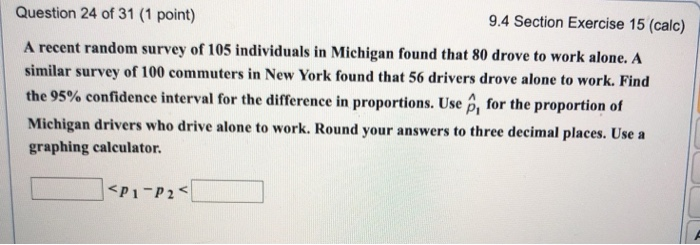Question 24 of 31 (1 point) 9.4 Section Exercise 15 (calc) A recent random survey of 105 individuals in Michigan found that 80 drove to work alone. A similar survey of 100 commuters in New York found that 56 drivers drove alone to work. Find the 95% confidence interval for the difference in proportions. Use ê, for the proportion of Michigan drivers who drive alone to work. Round your answers to three decimal places. Use a graphing calculator. <P1-P2

• ### Question 15 of 30 (1 point) View problem in a pop-up 7.3 Section Exercise 27 Call...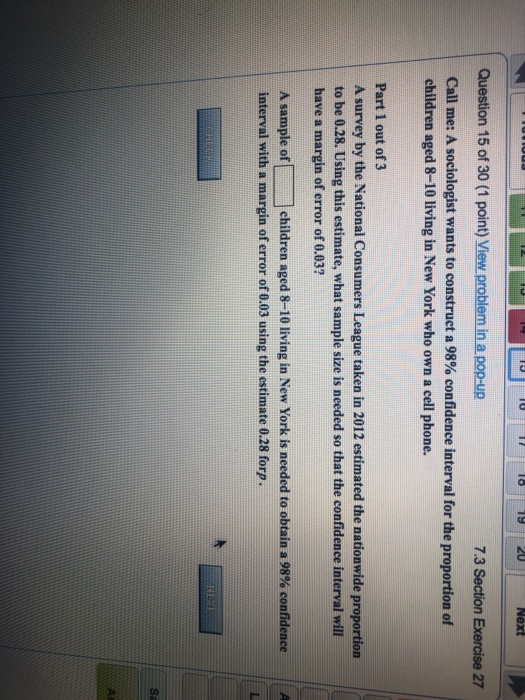Question 15 of 30 (1 point) View problem in a pop-up 7.3 Section Exercise 27 Call me: A sociologist wants to construct a 98% confidence interval for the proportion of children aged 8-10 living in New York who own a cell phone Part 1 out of 3 A survey by the National Consumers League taken in 2012 estimated the nationwide proportion to be 0.28. Using this estimate, what sample size is needed so that the confidence interval will have a...

• ### Question 2 of 5 (1 point) View problem in a pop-UR 8.2 Section Exercise 17-20 (table...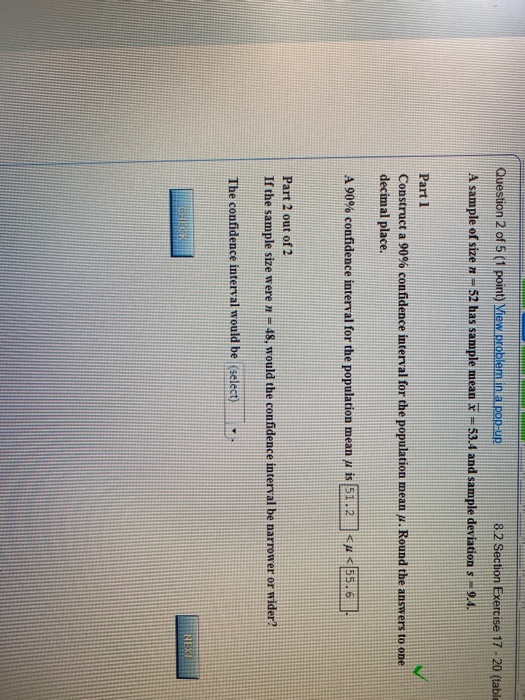Question 2 of 5 (1 point) View problem in a pop-UR 8.2 Section Exercise 17-20 (table A sample of size 52 has sample mean x = 53.4 and sample deviations -- 9.4. Part 1 Construct a 90% confidence interval for the population mean w. Round the answers to one decimal place. A 90% confidence interval for the population mean 4 is S.A. <<55.6 Part 2 out of 2 If the sample size were * = 48. would the confidence interval...

• ### Question 7 of 9 (1 point) View problem in a pop-up 7.1 Section Exercise 11 For...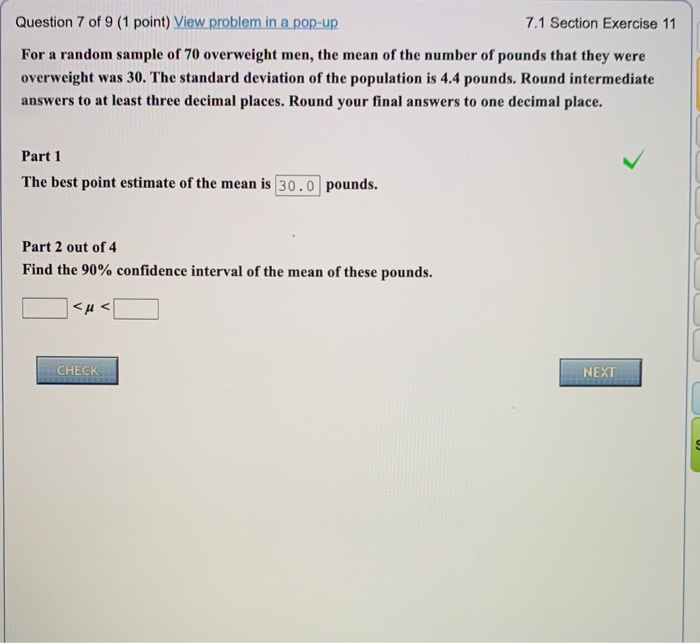Question 7 of 9 (1 point) View problem in a pop-up 7.1 Section Exercise 11 For a random sample of 70 overweight men, the mean of the number of pounds that they were overweight was 30. The standard deviation of the population is 4.4 pounds. Round intermediate answers to at least three decimal places. Round your final answers to one decimal place. Part 1 The best point estimate of the mean is 30.0 pounds. Part 2 out of 4 Find...

• ### Question 8 of 10 (2 points) Attempt 1 of 3 10.2 Section Exercise 11 Construct the...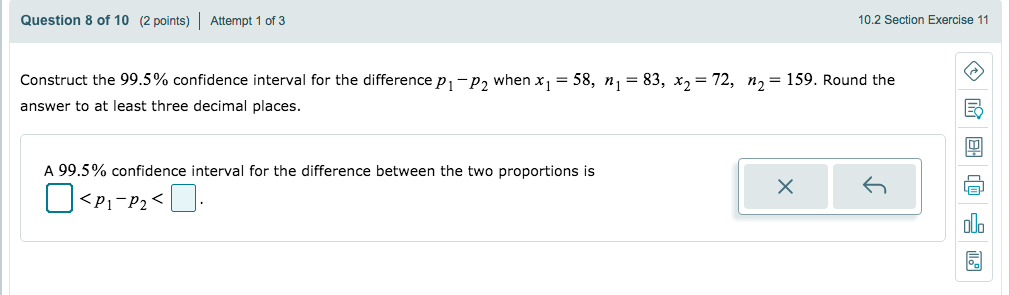Question 8 of 10 (2 points) Attempt 1 of 3 10.2 Section Exercise 11 Construct the 99.5% confidence interval for the difference Pi-p2 when x,-58, n,-83, x,-72, answer to at least three decimal places. n2-159. Round the 囤 A 99.5% confidence interval for the difference between the two proportions is P1-P2 alo

• ### Question 7 of 9 (1 point) View problem in a pop-up 7.1 Section Exercise 11 For...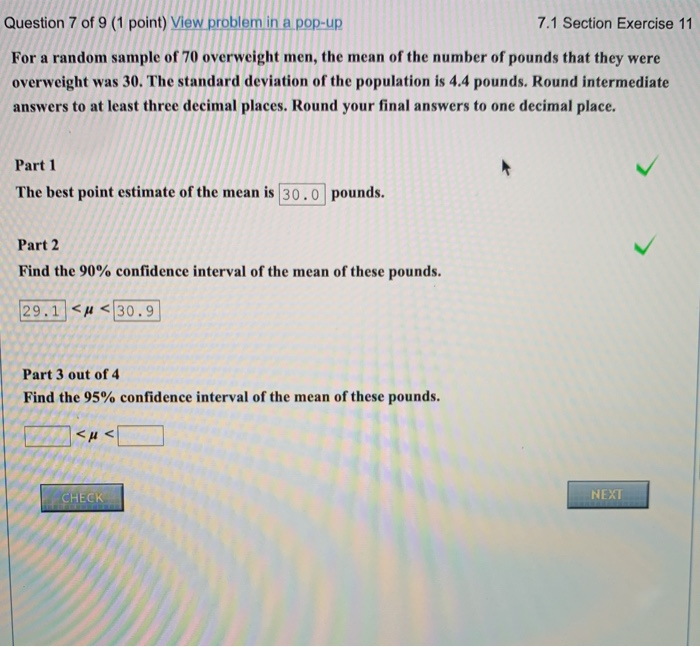Question 7 of 9 (1 point) View problem in a pop-up 7.1 Section Exercise 11 For a random sample of 70 overweight men, the mean of the number of pounds that they were overweight was 30. The standard deviation of the population is 4.4 pounds. Round intermediate answers to at least three decimal places. Round your final answers to one decimal place. Part 1 The best point estimate of the mean is 30.0 pounds. Part 2 Find the 90% confidence...

• ### Question 7 of 9 (1 point) View problem in a pop-up 7.1 Section Exercise 11 For...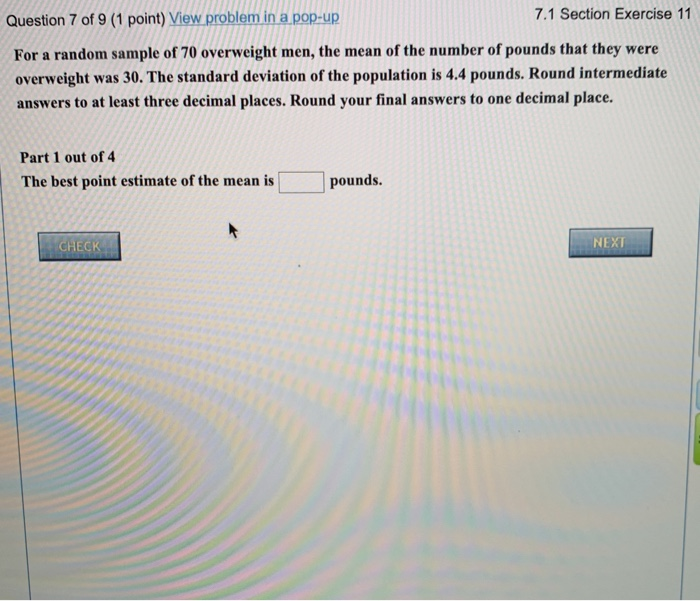Question 7 of 9 (1 point) View problem in a pop-up 7.1 Section Exercise 11 For a random sample of 70 overweight men, the mean of the number of pounds that they were overweight was 30. The standard deviation of the population is 4.4 pounds. Round intermediate answers to at least three decimal places. Round your final answers to one decimal place. Part 1 out of 4 The best point estimate of the mean is pounds. CHECK NEXT 7.1 Section...

• ### Question 2 of 3 (1 point) View problem in a pop-up 11.1 Section Exercise 8 (calc)...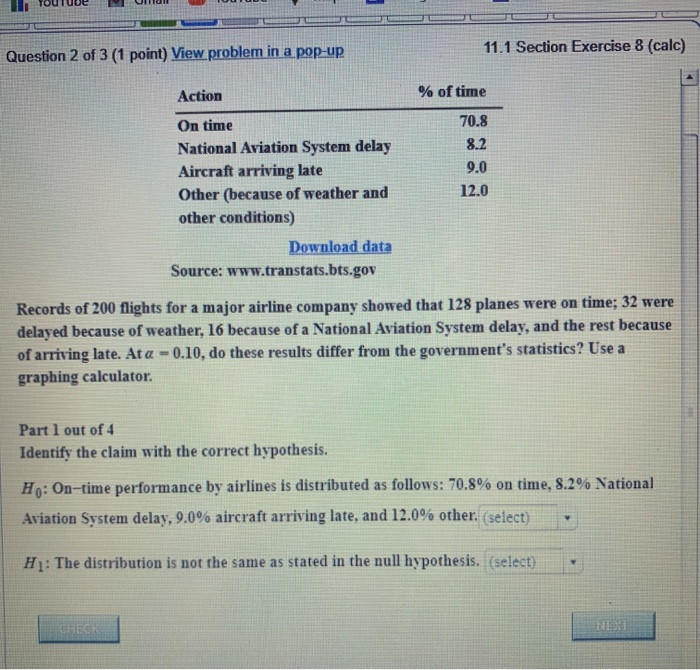Question 2 of 3 (1 point) View problem in a pop-up 11.1 Section Exercise 8 (calc) Action % of time 70.8 8.2 9.0 12.0 On time National Aviation System delay Aircraft arriving late Other (because of weather and other conditions) Download data Source: www.transtats.bts.gov Records of 200 flights for a major airline company showed that 128 planes were on time; 32 were delayed because of weather, 16 because of a National Aviation System delay, and the rest because of arriving...